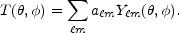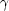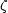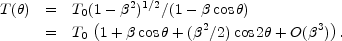### 2. DESCRIPTION OF CMB ANISOTROPIES

Observations show that the CMB contains anisotropies at the 10-5 level, over a wide range of angular scales. These anisotropies are usually expressed by using a spherical harmonic expansion of the CMB sky:The vast majority of the cosmological information is contained in the temperature 2 point function, i.e., the variance as a function of separation. Equivalently, the power per unit lnism |am|2 / 4.

The CMB has a mean temperature of T= 2.725 ± 0.001 K (1) , which can be considered as the monopole component of CMB maps, a00. Since all mapping experiments involve difference measurements, they are insensitive to this average level. Monopole measurements can only be made with absolute temperature devices, such as the FIRAS instrument on the COBE satellite . Such measurements of the spectrum are consistent with a blackbody distribution over more than three decades in frequency. A blackbody of the measured temperature corresponds to n= (2(3) /2) T3411 cm-3 and= (2 / 15) T44.64 × 10-34 g cm-30.260 eV cm-3.

The largest anisotropy is in the= 1 (dipole) first spherical harmonic, with amplitude 3.346 ± 0.017 mK . The dipole is interpreted to be the result of the Doppler shift caused by the solar system motion relative to the nearly isotropic blackbody field, as confirmed by measurements of the velocity field of local galaxies . The motion of an observer with velocity= v / c relative to an isotropic Planckian radiation field of temperature T0 produces a Doppler-shifted temperature patternAt every point in the sky, the spectrum is essentially blackbody, but the spectrum of the dipole is the differential of a blackbody spectrum, as confirmed by Ref. .

The implied velocity  for the solar system barycenter is v = 368 ± 2 km s-1, assuming a value T0 = T, towards (, b) = (263.85° ± 0.10°, 48.25° ± 0.04°). Such a solar system velocity implies a velocity for the Galaxy and the Local Group of galaxies relative to the CMB. The derived value is vLG = 627 ± 22 km s-1 toward (, b) = (276° ± 3°, 30° ± 3°), where most of the error comes from uncertainty in the velocity of the solar system relative to the Local Group.

The dipole is a frame dependent quantity, and one can thus determine the `absolute rest frame' of the Universe as that in which the CMB dipole would be zero. Our velocity relative to the Local Group, as well as the velocity of the Earth around the Sun, and any velocity of the receiver relative to the Earth, is normally removed for the purposes of CMB anisotropy study.

Excess variance in CMB maps at higher multipoles (2) is interpreted as being the result of perturbations in the energy density of the early Universe, manifesting themselves at the epoch of the last scattering of the CMB photons. In the hot Big Bang picture, this happens at a redshift z1100, with little dependence on the details of the model. The process by which the hydrogen and helium nuclei can hold onto their electrons is usually referred to as recombination . Before this epoch, the CMB photons are tightly coupled to the baryons, while afterwards they can freely stream towards us.

Theoretical models generally predict that the am modes are Gaussian random fields, and all tests are consistent with this simplifying assumption . With this assumption, and if there is no preferred axis, then it is the variance of the temperature field which carries the cosmological information, rather than the values of the individual ams; in other words the power spectrum infully characterizes the anisotropies. The power at eachis (2+ 1) C/ (4), where C<|am|2>, and a statistically isotropic sky means that all ms are equivalent. We use our estimators of the Cs to constrain their expectation values, which are the quantities predicted by a theoretical model. For an idealized full-sky observation, the variance of each measured C(the variance of the variance) is [2 / (2+ 1)] C2. This sampling uncertainty (known as cosmic variance) comes about because each Cis2 distributed with (2+ 1) degrees of freedom for our observable volume of the Universe. For partial sky coverage, fsky, this variance is increased by 1 / fsky and the modes become partially correlated.

It is important to understand that theories predict the expectation value of the power spectrum, whereas our sky is a single realization. Hence the `cosmic variance' is an unavoidable source of uncertainty when constraining models; it dominates the scatter at lowers, while the effects of instrumental noise and resolution dominate at highers.

There is no one-to-one conversion between the angle subtended by a particular wavevector projected on the sky and multipole. However, a single spherical harmonic Ym corresponds to angular variations of~/. CMB maps contain anisotropy information from the size of the map (or in practice some fraction of that size) down to the beam-size of the instrument,. One can think of the effect of a Gaussian beam as rolling off the power spectrum with the function e-(+1)2.

For less than full sky coverage, themodes are correlated. Hence, experimental results are usually quoted as a series of `band powers', defined as estimators of(+ 1) C/ 2over different ranges of. because of the strong foreground signals in the Galactic Plane, even `all-sky' surveys, such as COBE and WMAP involve a cut sky. The amount of binning required to obtain uncorrelated estimates of power also depends on the map size.